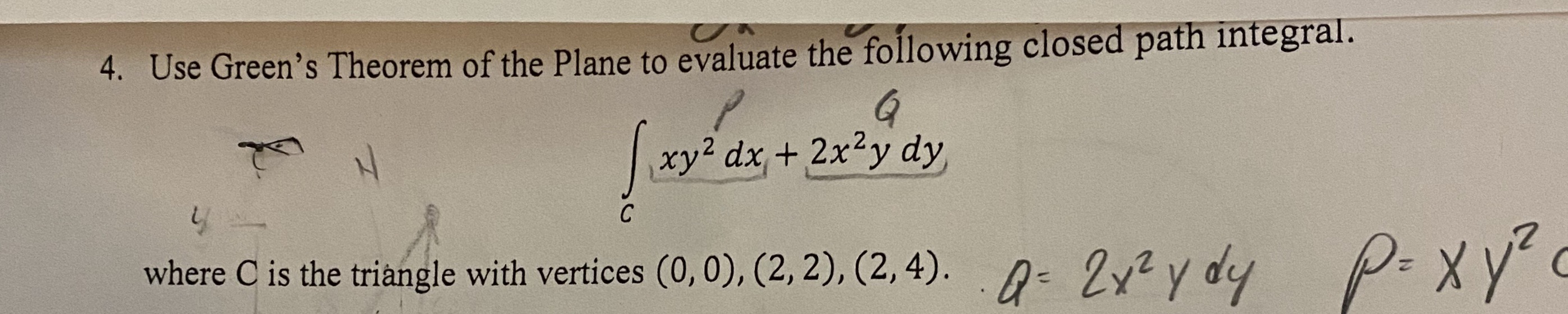# 4. Use Green's Theorem of the Plane to evaluate the following closed path integral.xy2 dx+ 2x2y dyСwhere C is the triangle with vertices (0,0), (2, 2), (2, 4). Zyy dyP.xyху

Question
8 viewshelp_outlineImage Transcriptionclose4. Use Green's Theorem of the Plane to evaluate the following closed path integral. xy2 dx+ 2x2y dy С where C is the triangle with vertices (0,0), (2, 2), (2, 4). Zyy dy P.xy ху fullscreen
check_circle

Step 1

According to green’s theorem closed path integral over the curve C is calculated by,

Step 2

Identify P and Q from the given integral.

Hence, find the partial derivatives.

Step 3

Apply green’...

### Want to see the full answer?

See Solution

#### Want to see this answer and more?

Solutions are written by subject experts who are available 24/7. Questions are typically answered within 1 hour.*

See Solution
*Response times may vary by subject and question.
Tagged in

### Math